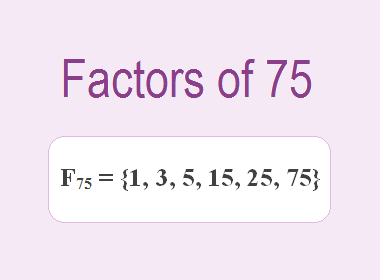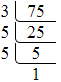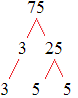# Factors of 75The factors of 75 are 1, 3, 5, 15, 25, and 75 i.e. F75 = {1, 3, 5, 15, 25, 75}. The factors of 75 are those numbers that can divide 75 without leaving a remainder.

We can check if these numbers are factors of 75 by dividing 75 by each of them. If the result is a whole number, then the number is a factor of 75. Let's do this for each of the numbers listed above:

·        1 is a factor of 75 because 75 divided by 1 is 75.

·        3 is a factor of 75 because 75 divided by 3 is 25.

·        5 is a factor of 75 because 75 divided by 5 is 15.

·        15 is a factor of 75 because 75 divided by 15 is 5.

·        25 is a factor of 75 because 75 divided by 25 is 3.

·        75 is a factor of 75 because 75 divided by 75 is 1.

Therefore, the factors of 75 are 1, 3, 5, 15, 25, and 75.********************

********************

## Prime Factorization of 75

Prime factorization is one of the applications of factors. Prime factorization is the process of finding the prime numbers that can multiply together to give the original number. To find the prime factorization of 75, we can start by dividing it by its smallest prime factor, which is 3. This gives us:

75 ÷ 3 = 25

So, 3 is a factor of 75 and 25 is a factor of 75. Next, we divide 25 by its smallest prime factor, which is 5. This gives us:

25 ÷ 5 = 5

So, 5 is a factor of 75. Now, we have found all the prime factors of 75, which are 3 and 5. We can write the prime factorization of 75 as:

75 = 3 × 5 × 5

Or, we can obtain the prime factors of 75 by the following procedure of division as given below:75 = 3 × 5 × 5

Or, we can obtain the prime factors of 75 by the Factor Tree method as given below:75 = 3 × 5 × 5

In conclusion, the factors of 75 are 1, 3, 5, 15, 25, and 75. These factors can be useful in various applications of mathematics, including prime factorization and solving equations.